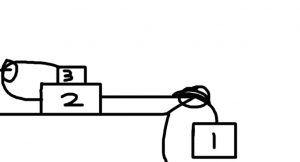# How to solve physics problems where multiple boxes are stacked on top of each other in a multiple pulley system, with friction

Let’s combine two concepts we know about: stacked boxes on top of each other (with friction) AND multiple pulley systems with boxes. Before tackling this problem, review stacked boxes physics and multiple pulley system problems. Once you have those taken care of, continue to our problem:

Example: Find the tension in both strings and the acceleration of the blocks as illustrated in the diagram below:The masses of objects 1, 2 and 3 are 40 kg, 10 kg, and 5 kg, respectively. There is a coefficient of static friction between block 2 and the floor (0.2) and another coefficient of static friction between box 3 and 2 (0.15).

Box 1 hangs down, connected to box 2. Box 2 is connected to box 3, but box 3 also lies on top of box 2 as well. Box 2 and 3 are affected by friction.

The basic premise is that we must develop 3 equations to solve for three unknowns, which will be the two tension cables and the common acceleration of the blocks in the system.

When making the equations be careful of your signs. Mass 1 will drop down, while there’s upward tension force. This causes box 2 to slide to the right (note, it might be possible for box 2 to not slide if it and box 3 are too heavy, but we purposely made box 1 heavier).

If box 2 slides right, there’s a friction force to the left. There’s also tension to the left of box 2. Box 3 has a friction to the right due to moving to the left (box 2 moves to the right), but note that when you have friction between two surfaces (two boxes), the friction is equal and opposite in direction for both boxes.

So if box 3 has friction to the right, box 2 has that same friction to the left as well! Box 3 has a tension as well.

Let’s make our equations. We call tension 1 the cable on the right, and tension 2 is the cable on the left.

Note that we make positive downward for the first box, make it positive in the direction the system moves in. For box 1, down is positive and up is negative. For box 2, right is positive and left is negative. For box 3, left is positive and right is negative. You don’t necessarily have to do it like that, you can make your own coordinate system, but be consistent.

For box 1:

${m_1}g – {T_1} = {m_1}a$

$392.4 – {T_1} = 40a$

For box 3:

${F_F} = {\mu _S}{F_N}$

${F_F} = {\mu _S}{m_3}g$

${T_2} – {\mu _S}{m_3}g = {m_3}a$

${T_2} – 7.36 = 5a$

And the last equation, the tricky box 2. Remember to get that friction term from the box above, both force tensions, and its own friction.

${T_1} – {T_2} – 7.36 – {\mu _S}({m_2} + {m_3})g = {m_2}a$

${T_1} – {T_2} – 7.36 – (0.2)(10 + 5)(9.81) = 10a$

${T_1} – {T_2} – 36.79 = 10a$

Note: Remember that the direction of the friction between boxes 2 and 3 are flipped depending on whether it’s box 2 or box 3 you’re looking at. The reason it ended up being negative in both equations was because we flipped our coordinate system between boxes, but it still correctly is in the opposite direction – the friction is to the right for box 3, and to the left for box 2.

Let’s sort out and simplify our 3 equations:

$-1{T_1} + 0{T_2} – 40a = -392.4$

$0{T_1} + 1{T_2} – 5a = 7.36$

$1{T_1} – 1{T_2} – 10a = 36.79$

We cast it into a matrix:

$\left[ {\begin{array}{*{20}{c}}1&0&{ – 40}\\0&1&{ – 5}\\1&{ – 1}&{ – 10}\end{array}} \right]\left[ {\begin{array}{*{20}{c}}{{T_1}}\\{{T_2}}\\a\end{array}} \right] = \left[ {\begin{array}{*{20}{c}}{392.4}\\{7.36}\\{36.79}\end{array}} \right]$

Solving, T1 is 139.13 N, T2 is 39.02 N, and acceleration is 6.33 m/s2.

Example: Use the diagram above again. The masses of objects 2 and 3 are 10 kg and 5 kg, respectively. The first mass 1 is now unknown. There is a coefficient of static friction between block 2 and the floor (0.2) and another coefficient of static friction between box 3 and 2 (0.15). How much does mass 1 need to be for the system to start moving?

For the system to start moving, acceleration a = 0. Now m1 is an unknown. Re-use most of the equations we made earlier:

$\begin{array}{l}{m_1}g – {T_1} = {m_1}a\\ – {T_1} + (9.81){m_1} = 0\\{T_2} – {\mu _S}{m_3}g = {m_3}a\\{T_2} = 7.36\\{T_1} – {T_2} – 7.36 – {\mu _S}({m_2} + {m_3})g = {m_2}a\\{T_1} – 7.36 – 7.36 – (0.2)(10 + 5)(9.81) = 0\\{T_1} = 44.15\\ – {T_1} + (9.81){m_1} = 0\\{m_1} = \frac{{44.15}}{{9.81}}\\{m_1} = 4.5\end{array}$

So the first block needs to be at least 4.5 kg for the system to start moving.

Why does it only take 4.5 kg to start to move 10+5 kg? Well gravity is pretty powerful, and the coefficients of frictions are not that high.

Try a physics problem with multiple pulleys involving the “conservation of string” concept